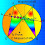## Tuesday, June 9, 2009

### Problem 300: Tangent to a circle, Secant

Proposed Problem
Click the figure below to see the complete problem 300 about Tangent to a circle, Secant.Complete Problem 300
Collection of Geometry Problems

Level: High School, SAT Prep, College geometry

1.This comment has been removed by a blog administrator.

2.This comment has been removed by the author.

3.Join BC, OA and draw FF’ & EE’ perpendicular to OA meeting AB in F’ and AC in E’ respectively. Now BCFF’ and EE’FF’ are both isosceles trapezia which are concyclic. Hence angle FF’B =angle E’EB. By the chord-secant theorem, angle F’BF=angle ECB=angle EE’B =angle E’BC. Therefore, angle E’BC + angle E’BF = angle E’BC + angle E’BF or angle E’BE = angle CBF. But the latter is =angle CF’F being angles in the same segment.In other words, angle CF’F =angle EBE’. This makes triangles FBF’ and EBE’ similar because of which, BE/FF’ =EE’/CF or a/FF’= EE’/b or EE’*FF’ = a*b ------------------(1)
We note that EF= E’F” =x and EF’ =E’F = (b –a) by our construction. –-------------(2)
Applying Ptolemy’s Theorem to EE’FF’ we obtain, EF*E’F’=EF’*E’F+EE’*FF’. Using (1) & (2) above, x^2 = (b-a)^2 + ab = a^2 + b^2 – ab. QED.
Ajit: ajitathle@gmail.com

4.Connect OA and BC and we see that ABC is an isosceles with OA dividing it into equal halves. Let G,H be the reflections of E and F over OA on the opposite sides.
Since AB=AC we can easily derive that GF=HE=b-a and CH=BF=a
Using alternate segment theorem, it can be easily seen that GBE similar to HFC
GE.HF=a.b
Also FHGE being isosceles trapeziod is concyclic
hence x^2=(b-a)2+GE.HF
=> x^2=a^2+b^2-a.b

5.Nice problem